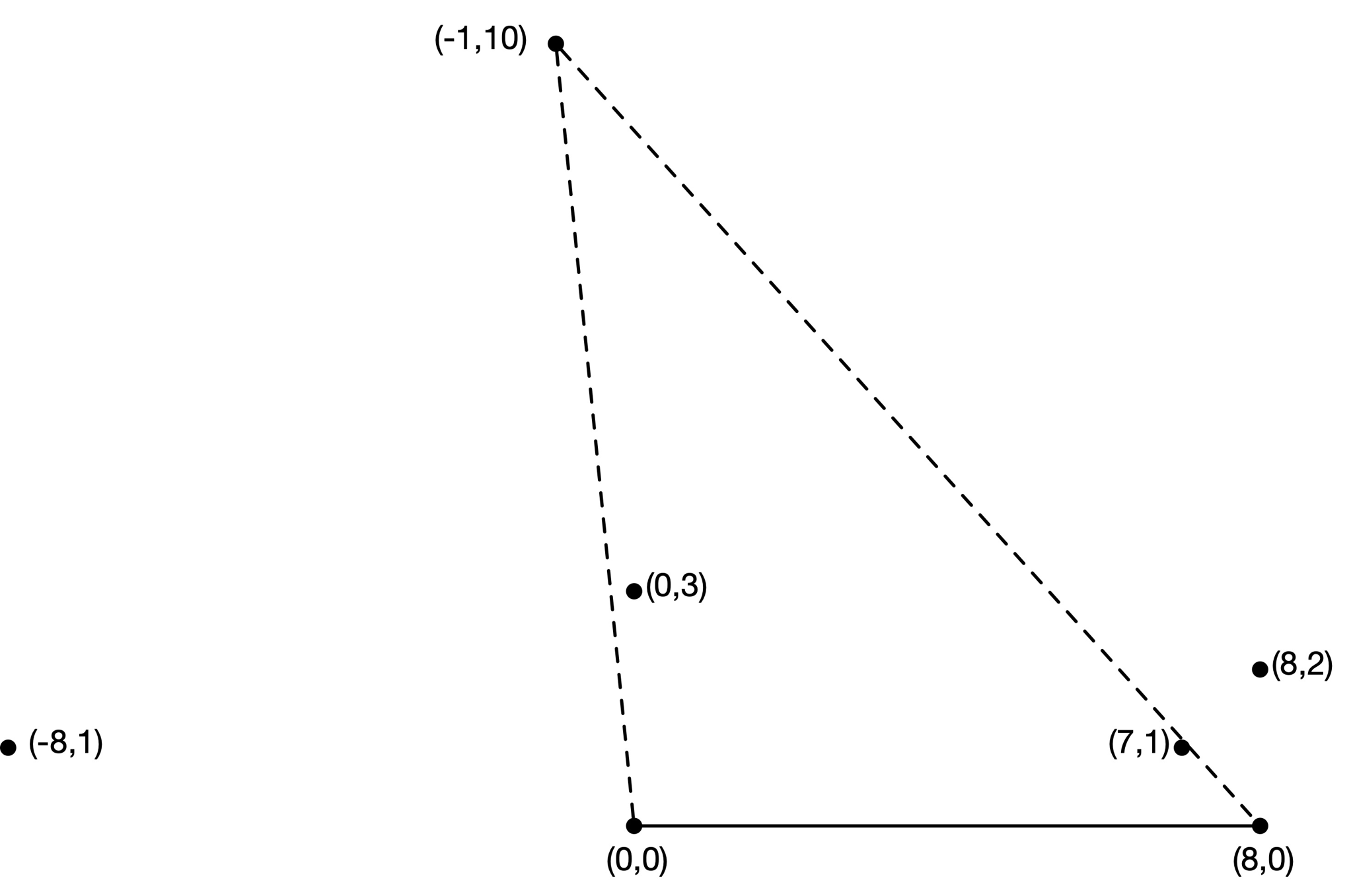시간 제한메모리 제한제출정답맞힌 사람정답 비율
4 초 1024 MB533100.000%

## 문제

You recently discovered there is treasure buried on your farm land. A lot of treasure! You quickly decide to put a fence around the land.

Alas, you have but a single fence post! You will have to drive to town to get more fencing material. But you can't just leave the land as open as it is, so you decide to create a makeshift fence to protect some of the treasure while you are gone. You will place the post in the ground and run some wire in a straight line between two sides of your barn wall and the fence post to section off a triangular area. Also, the ground is very hard: only places that were dug up to bury a treasure are soft enough for you to quickly place the fence post.

To figure out the best option, you first calculate the following. For each of the treasures in your field, if you were to place the fence post at that treasure and complete the fence as described, then what is the total value of all treasures that would be enclosed by the fence? Note that the treasure under the post you place is not considered enclosed by the fence (it might not be safe since someone could dig around the post).

Sample Input 1 is illustrated below. The triangle that includes the point $(-1,10)$ encloses exactly two other treasure points which have total value $4+8=12$.## 입력

The first line of input contains two integers $n$ ($1 \leq n \leq 10^5$) and $x$ ($1 \leq x \leq 10^9$), where $n$ is the number of treasure points and $x$ fixes the two corners of the barn wall at locations $(0,0)$ and $(x,0)$.

Each of the next $n$ lines contains three integers $x$, $y$, and $v$ ($-10^9 \leq x \leq 10^9$, $1 \leq y \leq 10^9$, and $1 \leq v \leq 10^9$) giving the location $(x,y)$ and value $v$ of one of the treasure points. All of these points are distinct. It is also guaranteed that for each point, the triangle formed with the barn wall will not contain any other treasure point on its boundary.

## 출력

Output $n$ lines, one for each treasure point in the order of the input. For each point output a single integer, which is the total value of all points in the interior of the triangle that point forms with the barn wall. Note that the value of the point itself should be excluded from this sum.

## 예제 입력 1

5 8
-8 1 1
-1 10 2
0 3 4
7 1 8
8 2 16


## 예제 출력 1

0
12
0
0
8


## 예제 입력 2

6 6
0 1 1
2 3 10
2 5 100
3 1 1000
3 5 10000
4 5 100000


## 예제 출력 2

0
1000
1010
0
1010
1000


## 출처• 문제를 만든 사람: Zachary Friggstad